Principles of Accounting, Volume 1: Financial Accounting

# 11.5Describe Some Special Issues in Accounting for Long-Term Assets

Principles of Accounting, Volume 1: Financial Accounting11.5 Describe Some Special Issues in Accounting for Long-Term Assets

A company will account for some events for long-term assets that are less routine than recording purchase and depreciation or amortization. For example, a company may realize that its original estimate of useful life or salvage value is no longer accurate. A long-term asset may lose its value, or a company may sell a long-term asset.

### Revision of Remaining Life or Salvage Value

As you have learned, depreciation is based on estimating both the useful life of an asset and the salvage value of that asset. Over time, these estimates may be proven inaccurate and need to be adjusted based on new information. When this occurs, the depreciation expense calculation should be changed to reflect the new (more accurate) estimates. For this entry, the remaining depreciable balance of the net book value is allocated over the new useful life of the asset. To work through this process with data, let’s return to the example of Kenzie Company.

• Kenzie has a press worth $58,000. • Its salvage value was originally estimated to be$10,000.
• Its economic life was originally estimated to be five years.
• Kenzie uses straight-line depreciation.

After three years, Kenzie determines that the estimated useful life would have been more accurately estimated at eight years, and the salvage value at that time would be $6,000. The revised depreciation expense is calculated as shown: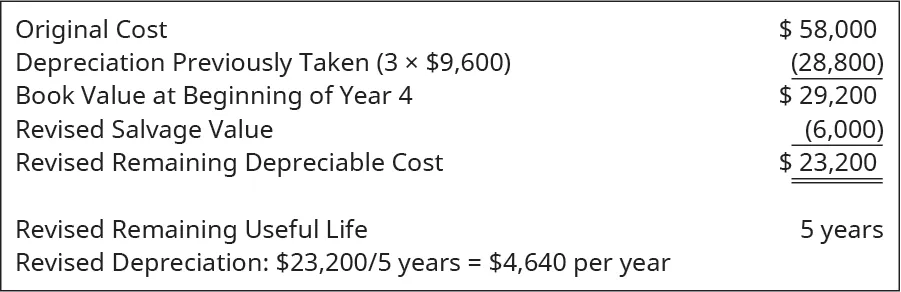These revised calculations show that Kenzie should now be recording a depreciation of$4,640 per year for the next five years.

#### Useful Life

Georgia-Pacific is a global company that employs a wide variety of property, plant, and equipment assets in its production facilities. You work for Georgia-Pacific as an accountant in charge of the fixed assets subsidiary ledger at a warehouse facility in Pennsylvania. You find out that the useful lives for the fork trucks need to be adjusted. As an asset category, the trucks were bought at the same time and had original useful lives of seven years. However, after depreciating them for two years, the company makes improvements to the trucks that allow them to be used outdoors in what can be harsh winters. The improvements also extend their useful lives by two additional years. What is the remaining useful life after the improvements?

Solution

Seven original years – two years depreciated + two additional years = seven years remaining.

### Obsolescence

Obsolescence refers to the reduction in value and/or use of the asset. Obsolescence has traditionally resulted from the physical deterioration of the asset—called physical obsolescence. In current application—and considering the role of modern technology and tech assets—accounting for functional obsolescence is becoming more common. Functional obsolescence is the loss of value from all causes within a property except those due to physical deterioration. With functional obsolescence, the useful life still needs to be adjusted downward: although the asset physically still works, its functionality makes it less useful for the company. Also, an adjustment might be necessary in the salvage value. This potential adjustment depends on the specific details of each obsolescence determination or decision.

### Sale of an Asset

When an asset is sold, the company must account for its depreciation up to the date of sale. This means companies may be required to record a depreciation entry before the sale of the asset to ensure it is current. After ensuring that the net book value of an asset is current, the company must determine if the asset has sold at a gain, at a loss, or at book value. We look at examples of each accounting alternative using the Kenzie Company data.

Recall that Kenzie’s press has a depreciable base of $48,000 and an economic life of five years. If Kenzie sells the press at the end of the third year, the company would have taken three years of depreciation amounting to$28,800 ($9,600 × 3 years). With an original cost of$58,000, and after subtracting the accumulated depreciation of $28,800, the press would have a book value of$29,200. If the company sells the press for $31,000, it would realize a gain of$1,800, as shown.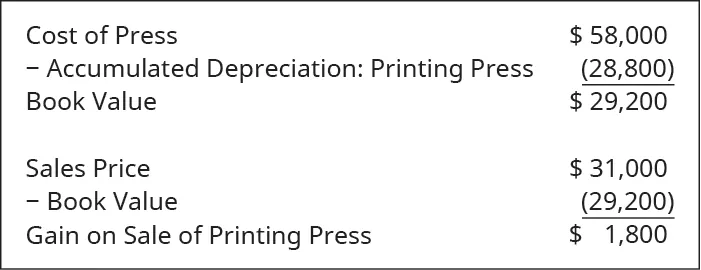The journal entry to record the sale is shown here.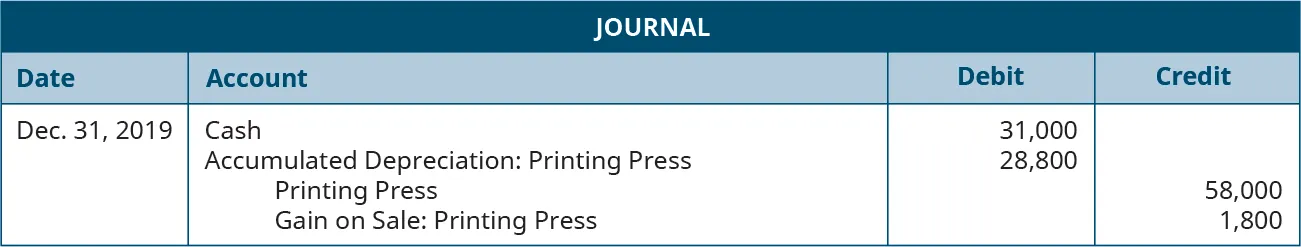If Kenzie sells the printing press for $27,100, what would the journal entries be? The book value of the press is$29,200, so Kenzie would be selling the press at a loss. The journal entry to record the sale is shown here.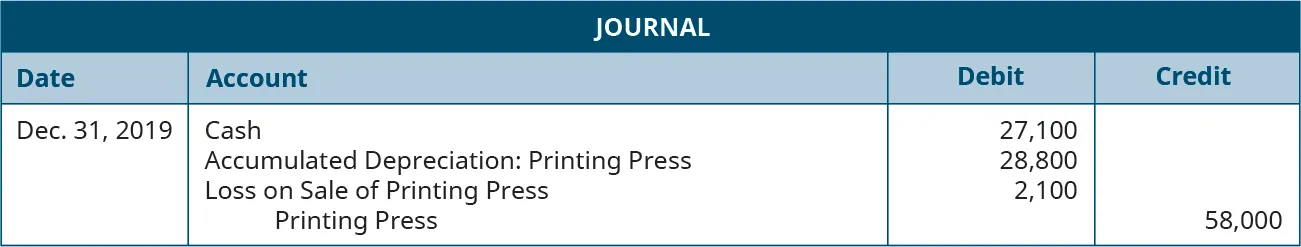What if Kenzie sells the press at exactly book value? In this case, the company will realize neither a gain nor a loss. Here is the journal entry to record the sale.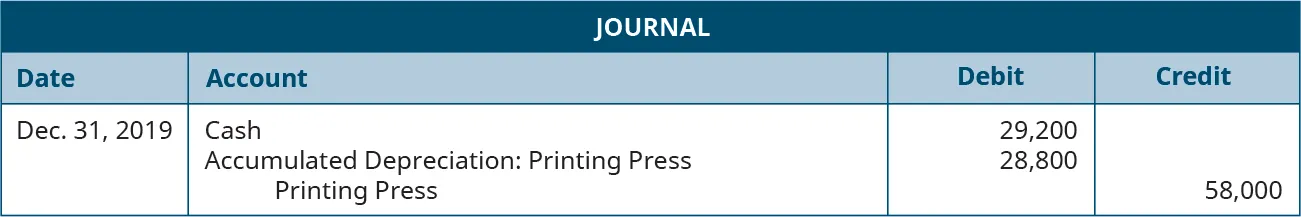While it would be ideal to estimate a salvage value that provides neither a gain nor a loss upon the retirement and sale of a long-term asset, this type of accuracy is virtually impossible to reach, unless you negotiate a fixed future sales price. For example, you might buy a truck for $80,000 and lock in a five-year life with 100,000 or fewer miles driven. Under these conditions, the dealer might agree to pay you$20,000 for the truck in five years.

Under these conditions, you could justify calculating your depreciation over a five-year period, using a depreciable base of $60,000. Under the straight-line method, this would provide an annual depreciation amount of$12,000. Also, when you sell the truck to the dealer after five years, the sales price will be $20,000, and the book value will be$20,000, so there would be neither a gain nor a loss on the sale.

In the Kenzie example where the asset was sold for $31,000 after three years, Kenzie should have recorded a total of$27,000 in depreciation (cost of $58,000 less the sales value of$31,000). However, the company recorded $28,800 in depreciation over the three-year period. Subtracting the gain of$1,800 from the total depreciation expense of $28,800 shows the true cost of using the asset as$27,000, and not the depreciation amount of $28,800. When the asset was sold for$27,100, the accounting records would show $30,900 in depreciation (cost of$58,000 less the sales price of $27,100). However, depreciation is listed as$28,800 over the three-year period. Adding the loss of $2,100 to the total depreciation expense of$28,800 results in a cost of $30,900 for use of the asset rather than the$28,800 depreciation.

If the asset sells for exactly the book value, its depreciation expense was estimated perfectly, and there is no gain or loss. If it sells for $29,200 and had a book value of$29,200, its depreciation expense of \$28,800 matches the original estimate.

### Think It Through

#### Depreciation of Long-Term Assets

You are a new staff accountant at a large construction company. After a rough year, management is seeking ways to minimize expenses or increase revenues before year-end to help increase the company’s earnings per share. Your boss has asked staff to think “outside the box” and has asked to you look through the list of long-term assets to find ones that have been fully depreciated in value but may still have market value. Why would your manager be looking for these specific assets? How significantly might these items impact your company’s overall performance? What ethical issues might come into play in the task you have been assigned?

Order a print copy

As an Amazon Associate we earn from qualifying purchases.